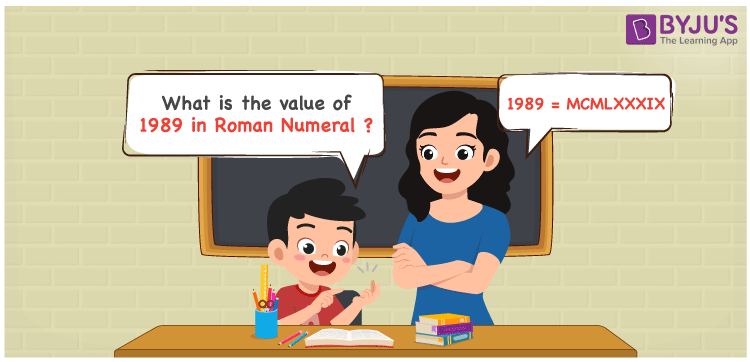# 1989 in Roman Numerals

1989 in Roman Numerals is MCMLXXXIX. Any Roman numeral uses only the 7 primary symbols which are I, V, X, L, C, D, and M, Where M represents 1000, C represents 100, L represents 50, X represents 10 and I represents 1. MCMLXXXIX represents the number 1989 which is obtained by the addition and subtraction rules of Roman Numerals. What are Roman numerals? can be referred for more details.

 Number Roman Numeral 1989 MCMLXXXIX

## How to Write 1989 in Roman Numerals?To convert 1989 in Roman Numerals, we need to represent 1989 as the sum of the fundamental symbols. 1989 can be broken down as

1. The number 1000,
2. Difference of 1000 and 100 to get 900
3. Add 50 to thrice of 10 to get 80
4. Difference of 10 and 1 to get 9.
5. Add all the numbers from previous steps.

Let us understand it with Numerical representation.

1989 = 1000 + 900 + 80 + 9

1989 = 1000 + (1000 – 100) + (50 + 10 + 10 + 10) + (10 – 1)

1989 = M + (M – C) + (L + X + X + X) + (X – I)

1989 = M + (CM) + LXXX + (IX)

1989 = MCMLXXXIX.

## Video Lesson on Roman Numerals## Frequently Asked Questions on 1989 in Roman Numerals

Q1

### How to write the number 1989 in Roman Numerals?

1989 in Roman Numerals is written as MCMLXXXIX.
Q2

### Does MCMLXXXIX represent Roman Numerals?

Yes. MCMLXXXIX represents Roman Numerals and it is the number 1989
Q3

### What is the year 1989 in Roman numerals?

The year 1989 in Roman Numerals is MCMLXXXIX.